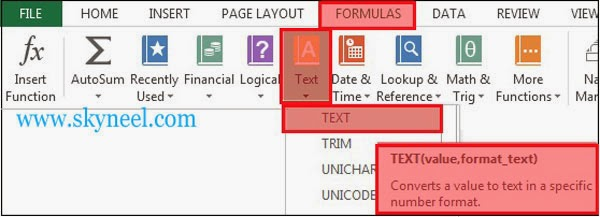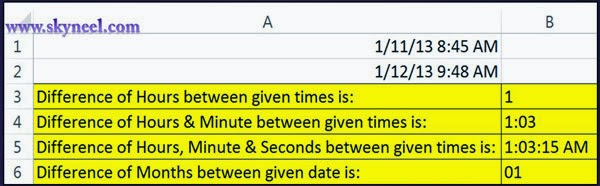# Difference between two given times in excel

Difference between two given times, using text function in excel. Text function change a value to text in a specific number format.

How to calculate the difference between 2 given times, using text function in excel. We are facing this problem lot of time in excel. In that case you have to take following some simple steps in excel.  You can use text function in this post. Text function is very important and popular function of Microsoft Excel. This functions is used to change any given value into the text in a given special number format.

### Syntax of text function:

TEXT(value,format_text)
Value:   It is any number, a formula that calculates to a number value, or a address to a cell having a number value.

Format_text: It is a number format as a text value which is surrounded in double quotation marks. There are lot of number format like date, time, general, number, currency etc. in the “Number” tab from the “Format cells” dialog box.

For example: If you want calculate difference between two times to view or print number of “hours”, number of “minutes” and number of “seconds” in Microsoft Excel. You have to take following steps to find the difference between two times.

## Calculate the difference:

Step 1.  You have to type given times in cell A1 and A2 in given
format.     Cell A1=1/11/2013  8:45:25 AM     Cell A2= 1/12/2013  9:48:40 AM

Step 2.  If you want to change the format of given time in that case you have to click on the Home tab > click “Number” dialog box launcher button > this will open “Format Cells” dialog box ->  Select Date in category -> then select this given format from the list  (3/4/01 1:30 PM) then click OK.Step 3. Type following given formulas in specific cells to get the result Cell A3: If you want to calculate number of hours only between two given times:

=TEXT(A2-A1,”h”)                Result is =1

Cell A4: If you want to calculate hours & minutes both between
two given times:

=TEXT(A2-A1,”h:mm”)             Result is =1:03Cell A5: If you want to calculate hours, minutes and seconds between two given times:=TEXT(A2-A1,”h:mm:s AM/PM “)     Result is=1:03:15 AM

Cell A6:  If you want to calculate months between two given date & times: =TEXT(A2-A1,”mm”) Result is =01

I hope you like this Difference between two given times in excel guide. Give your important suggestions in the comment box and also share this article to improve your knowledge with your friends, staff member and many more. Thanks.